Open In App

# Functions in Discrete Mathematics

Functions are an important part of discrete mathematics. This article is all about functions, their types, and other details of functions. A function assigns exactly one element of a set to each element of the other set. Functions are the rules that assign one input to one output. The function can be represented as f: A B. A is called the domain of the function and B is called the codomain function

### Functions:

• A function assigns exactly one element of one set to each element of other sets.
• A function is a rule that assigns each input exactly one output.
• A function f from A to B is an assignment of exactly one element of B to each element of A (where  A and B are non-empty sets).
• A function f from set A to set B is represented as f: A ⇢ B where A is called the domain of f and B is called as codomain of f.
• If b is a unique element of B to element a of A assigned by function F then, it is written as f(a) = b.
• Function f maps A to B means f is a function from A to B i.e. f: A B

### Domain of a function:

• If f is a function from set A to set B then, A is called the domain of function f.
• The set of all inputs for a function is called its domain.

### Codomain of a function:

• If f is a function from set A to set B then, B is called the codomain of function f.
• The set of all allowable outputs for a function is called its codomain.

### Pre-image and Image of a function:

A function f: A B such that for each a ∈ A, there exists a unique b ∈ B such that (a, b) ∈ R then, a is called the pre-image of f and b is called the image of f.

### One-One function ( or Injective Function):

A function in which one element of the domain is connected to one element of the codomain.

A function f: A B is said to be a one-one (injective) function if different elements of A have different images in B.

f: A ⇢ B is one-one

⇒  a ≠ b ⇒  f(a) ≠ f(b)           for all a, b ∈  A

⇒  f(a) = f(b) ⇒ a = b           for all a, b ∈  A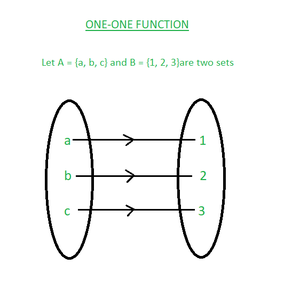ONE-ONE FUNCTION

### Many-One function:

A function f: A B is said to be a many-one function if two or more elements of set A have the same image in B.

A function f: A B is a many-one function if it is not a one-one function.

f: A ⇢ B is many-one

⇒ a ≠ b but f(a) = f(b)      for all a, b ∈  A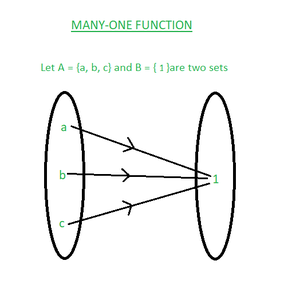MANY-ONE FUNCTION

### Onto function( or Surjective Function):

A function f: A -> B is said to be onto (surjective) function if every element of B is an image of some element of A  i.e. f(A) = B or range of f is the codomain of f.

A function in which every element of the codomain has one pre-image.

f: A ⇢ B is onto if for each b∈ B, there exists a∈ A such that f(a) = b.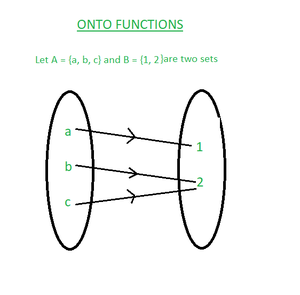ONTO FUNCTION

### Into Function:

A function f: A B is said to be an into a function if there exists an element in B with no pre-image in A.

A function f: A B is into function when it is not onto.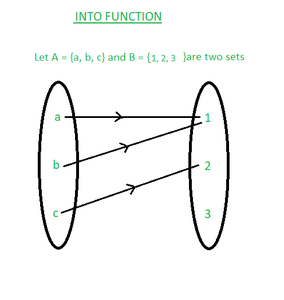INTO FUNCTION

### One-One Correspondent function( or  Bijective Function or One-One Onto Function):

A function which is both one-one and onto (both injective and surjective) is called one-one correspondent(bijective) function.

f : A ⇢ B is one-one correspondent (bijective) if:

• one-one i.e. f(a) = f(b) ⇒ a = b           for all a, b ∈  A
• onto i.e.  for each b ∈ B, there exists a ∈ A such that f(a) = b.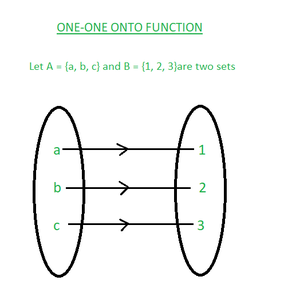ONE-ONE CORRESPONDENT FUNCTION

### One-One Into function:

A function that is both one-one and into is called one-one into function.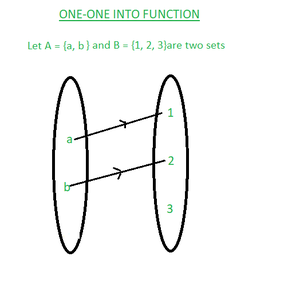ONE-ONE INTO FUNCTION

### Many-one onto function:

A function that is both many-one and onto is called many-one onto function.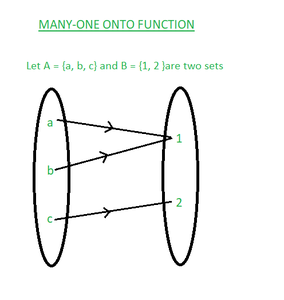MANY-ONE ONTO FUNCTION

### Many-one into a function:

A function that is both many-one and into is called many-one into function.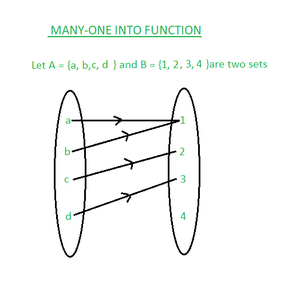MANY-ONE INTO FUNCTION

### Inverse of a function:

Let f: A B be a bijection then, a function g: B A which associates each element b ∈ B to a different element a ∈ A such that f(a) = b is called the inverse of f.

f(a) = b  ↔︎  g(b) = a

### Composition of functions :-

Let f: A B and g: B C be two functions then, a function gof: A C is defined by

(gof)(x) = g(f(x)), for all x ∈ A

is called the composition of f and g.

### Note:

Let X and Y be two sets with m and n elements and a function is defined as f : X->Y then,

• Total number of functions = nm
• Total number of one-one function = nPm
• Total number of onto functions = nmnC1(n-1)m + nC2(n-2)m – ………….. + (-1)n-1nCn-11m    if m ≥ n.

For the composition of functions f and g be two functions :

• fog ≠ gof
• If f and g both are one-one function then fog is also one-one.
• If f and g both are onto function then fog is also onto.
• If f and fog both are one-one function then g is also one-one.
• If f and fog both are onto function then it is not necessary that g is also onto.
• (fog)-1 = g-1o f-1
• f-1o f = f-1(f(a)) = f-1(b) = a
• fof-1 = f(f-1(b)) = f(a) = b

### Sample Questions:

Ques 1: Show that the function f : R ⇢ R, given by f(x) = 2x, is one-one and onto.

Sol:   For one-one:

```Let a, b ∈ R such that f(a) = f(b) then,
f(a) = f(b)
⇒ 2a = 2b
⇒ a = b
Therefore, f: R ⇢ R is one-one.```

For onto:

```Let p be any real number in R (co-domain).
f(x) = p
⇒ 2x = p
⇒ x = p/2
p/2 ∈ R for p ∈ R such that f(p/2) = 2(p/2) = p
For each p∈ R (codomain) there exists x = p/2 ∈ R (domain) such that f(x) = y
For each element in codomain has its pre-image in domain.
So, f: R ⇢ R is onto.
Since f: R ⇢ R is both one-one and onto.
f : R ⇢ R is one-one correspondent (bijective function).```

Ques 2: Let  f : R ⇢ R ; f(x) = cos x and  g : R ⇢ R ; g(x) = x3 . Find fog and gof.

Sol: Since the range of f is a subset of the domain of g and the range of g is a subset of the domain of f. So, fog and gof both exist.

```gof (x) = g(f(x)) = g(cos x) = (cos x)3 = cos3x
fog (x) = f(g(x)) = f(x3) = cos x3 ```

Ques 3: If f : Q ⇢ Q is given by f(x) = x2 , then find f-1(16).

Sol:

```Let f-1(16) = x
f(x) = 16
x2 = 16
x = ± 4
f-1(16) = {-4, 4}```

Ques 4 :- If f : R ⇢ R; f(x) = 2x + 7  is a bijective function then, find the inverse of f.

Sol: Let x ∈ R (domain), y ∈ R (codomain) such that f(a) = b

```f(x) = y
⇒ 2x + 7 = y
⇒ x = (y -7)/2
⇒ f-1(y) = (y -7)/2
Thus,  f-1 : R ⇢ R is defined as f-1(x) = (x -7)/2  for all x∈ R.```

Ques 5: If f : A ⇢ B and |A| = 5 and |B| = 3 then find total number of functions.

Sol: Total number of functions = 35 = 243